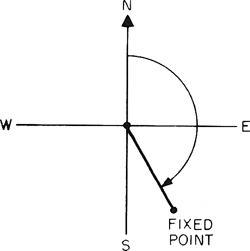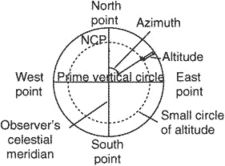azimuth

(redirected from Azimuthal angle)
Also found in: Dictionary, Thesaurus.
Related to Azimuthal angle: true azimuth

azimuth

(ăz`əməth), in astronomy, one coordinate in the altazimuth coordinate systemaltazimuth coordinate system
or horizon coordinate system,
astronomical coordinate system in which the position of a body on the celestial sphere is described relative to an observer's celestial horizon and zenith.
. It is the angular distance of a body measured westward along the celestial horizon from the observer's south point.

azimuth

(az -ă-mŭth) See horizontal coordinate system.
Collins Dictionary of Astronomy © Market House Books Ltd, 2006

Azimuth

(religion, spiritualism, and occult)

One can locate a specific celestial object in several ways, most of which involve specifying two coordinates. The azimuth is one of the coordinates of such a system. Although the notion of azimuth is basically simple, it is not simple to explain. Imagine that a group of people are looking at a star. From where they are standing, they can measure the angle between the horizon and the star. This gives them one coordinate in terms of angular distance (called the altitude, for obvious reasons). Then imagine a geometric plane that, like some kind of gigantic wall, cuts through Earth, intersecting the north and south poles, the place where they are standing, and the point directly over their heads (the zenith). They then measure another angle with their surveying instrument, this time between the imaginary wall and the star. This angular distance gives them the azimuth.

Sources:

Filbey, John, and Peter Filbey. The Astrologer’s Companion. Wellingborough, Northamptonshire, UK: Aquarian Press, 1986.
Gettings, Fred. Dictionary of Astrology. London: Routledge & Kegan Paul, 1985.

azimuth

[′az·ə·məth]
(astronomy)
Horizontal direction of a celestial point from a terrestrial point, expressed as the angular distance from a reference direction, usually measured from 0° at the reference direction clockwise through 360°.
(engineering)
In directional drilling, the direction of the face of the deviation tool with respect to magnetic north.
(geodesy)
Horizontal direction on the earth's surface.
McGraw-Hill Dictionary of Scientific & Technical Terms, 6E, Copyright © 2003 by The McGraw-Hill Companies, Inc.

azimuthazimuth
In plane surveying, a horizontal angle measured clockwise from north meridian to the direction of an object or fixed point.
McGraw-Hill Dictionary of Architecture and Construction. Copyright © 2003 by McGraw-Hill Companies, Inc.

azimuthi. A direction expressed as a horizontal angle, usually in degrees, measured clock-wise from a reference datum or direction, usually north. The azimuth will be a true zenith, grid azimuth, magnetic azimuth, or relative azimuth, depending upon which reference datum is used.
ii. The arc of the observer's rational horizon or the angle at his zenith contained between the observer's celestial meridian and the vertical circle through that body. It is the distance, measured in degrees, along the horizon westward from the south point of the horizon to the place where the vertical circle through an object intersects the horizon.
iii. As it pertains to aerial photography, the azimuth of a photograph is the clock-wise horizontal angle measured about the ground nadir point from the ground survey north meridian to the principal plane of the photograph. Also called azimuth of the principal plane.

azimuth

Astronomy navigation the angular distance usually measured clockwise from the north point of the horizon to the intersection with the horizon of the vertical circle passing through a celestial body
Collins Discovery Encyclopedia, 1st edition © HarperCollins Publishers 2005

azimuth

The trajectory of an angle measured in degrees going clockwise from a base point. A disk azimuth alignment test checks for the correct positioning of the read/write head to the track.
Copyright © 1981-2019 by The Computer Language Company Inc. All Rights reserved. THIS DEFINITION IS FOR PERSONAL USE ONLY. All other reproduction is strictly prohibited without permission from the publisher.
References in periodicals archive ?
(a) Schematic of slective emitter for free-space wave vector [k.sub.0], incident angle [theta], and azimuthal angle [phi] side length a, height h, and period p; (b) Simulated normal emittance for 2D Ta square array (red curve) and the bare Ta slab (blue curve); (c) normalized thermal emittance of our selective emitter (red curve), the bare Ta slab (blue curve), and the blackbody (gray curve) at 2000K.
Caption: Figure 9: Dependence between intensity of (111) reflection of [alpha](Al) solid solution of ECAPed alloy and azimuthal angle of the Debye-Scherrer ring.
The second event selection criterion is based on the correlations between relative azimuthal angles of photon interactions recorded in the detector in cases of three interactions observed in close time coincidence.
Finally, [DELTA][[phi].sub.i]; is the azimuthal angle covered by the area [A.sub.i], as seen from the center of the rectangular prism.
However, in this paper, the different scatterers are usually at the same azimuthal angle and may be of the same range at some scans (i.e., the range trajectories may be intersected).
Caption: Figure 2: RHEED patterns of the Si surface after growth of, respectively, (a) 100 nm, (b) 500 nm, and (c) 1000 nm Si film on Si(100), at the  azimuthal angle.
Variations of correction factor in longitudinal profile against azimuthal angle [theta] are given Figure 9, where about 6% increase of the load is observed with increased curvatures.
As the indices of the matrix we used the [??] angle to represent the rotation on the z axis (polar angle) and the [phi] angle for the rotation on the x-y-plane (azimuthal angle).
As a general trend, arises such that the muon transmission rate assumes a minimum value at an azimuthal angle of 166 mrad and a maximum value between 277 and 300 mrad.
If O is the origin of the x,y, z axes, (t) the trisector line x = y = z of the positive octant and n the plane x + y + z = 0 passing through the origin (O) and perpendicular to (t), then the tricomplex number u can be described by the projection S of the segment OP along the line (t), by the distance D from P to the line (t), and by the azimuthal angle 0 in the n plane.
The deposition rate was measured by a quartz crystal deposition rate controller (Sigma Instruments, SQM-160, USA) positioned close to the substrate and at almost the same azimuthal angle as that of the substrate.
We assume that the flow is axisymmetric (in spherical coordinates (r, [theta], [phi]), axisymmetric flow means v = [v.sub.r](r, [theta])[e.sub.r] + [v.sub.[theta]](r, [theta])[e.sub.[theta]], where [phi] is the azimuthal angle and [v.sub.[phi]] = 0, [partial derivative]/[partial derivative][phi] = 0) in which the flow quantities do not depend upon the azimuthal angle [phi].

Site: Follow: Share:
Open / Close